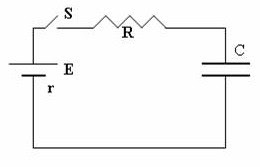## Pages

`“Life is like riding a bicycle.  To keep your balance you must keep moving.”–Albert Einstein`

## Saturday, December 11, 2010

### AP Physics C - Multiple Choice Practice Questions on Transients in RC and RL circuits

“Nearly every man develops an idea, works it up to the point where it looks impossible, and then he gets discouraged. That’s not the place to become discouraged.”
– Thomas A. Edison
Electric transient phenomena associated with circuits containing capacitors and resistors (CR circuits) are important from theoretical as well as practical point of view and they find place in the AP Physics C course. Transient phenomena associated with circuits containing inductors and resistors (LR circuits) are not as important as those associated with CR circuits but you should not ignore them.
Today we will discuss some multiple choice practice questions in this section. It will be a good idea to get yourself equipped sufficiently for the exercise by referring to my on this site.
Here are the questions with their solution:(1) In the circuit shown the the battery has emf E and internal resistance r. The switch S is closed at time t = 0. If the voltage across the capacitor is 90% of the emf E of the battery after time t’, what is the value of t’?
(a) RC ln10
(b) e–0.9/RC
(c) e0.9/RC
(d) (R+r)C ln(0.9)
(e) (R+r)C ln10
When a capacitor of capacitance C farad is charged by connecting it in series with a resistance R ohm and a battery of emf V volt, the charge Q coulomb on the capacitor after a time t seconds is given by
Q =Q0 (1 e–t/RC) where Q0 is the final (maximum) charge and ‘e’ is the base of natural logarithms.
Since the voltage V across the capacitor is Q/C, it follows that
V =V0 (1 e–t/RC) where V0 is the final (maximum) voltage which is the emf E of the battery.
Therefore we have
0.9 E = E[1 e–t’/(R+r)C] since the total resistance in the circuit is R+r.
This gives e–t/(R+r)C = 0.1
Or, et/(R+r)C = 10 from which t’ = (R+r)C ln10(2) The adjoining figure shows a resistance R connected in series with an inductance L and a battery of emf E through a switch S. The internal resistance of the battery is negligible. Which one of following graphs represents the nature of variation of the potential drop V across the resistance with time?If an inductor of inductance L and a resistor of resistance R are connected in series with a battery, the current I in the circuit increases exponentially with time and is given by
I = I0 (1 e–Rt/L) where I0 is the final (maximum) current in the circuit.
This shows that the current at time t = 0 (immediately after closing the switch) is zero. As time t increases, the current increases exponentially and reaches the final maximum value I0 (= E/R) when t becomes infinite.
As V = IR the potential drop V across R also increases exponentially with time as indicated by graph (a).(3) A capacitor C charged to V0 volt is discharged through the series combination of two resistances R ohm and 2R ohm by closing the switch S (Fig.). The base of natural logarithms is e. What is the current in the circuit when 3RC seconds are elapsed after closing the switch S?
(a) V0/(RC)
(b) V0/(3RC)
(c) V0/(3R)
(d) V0/(RCe)
(e) V0/(3Re)
The time constant of the circuit is 3RC and hence the voltage across the capacitor after 3RC seconds must be V0/e. You can write this if you remember how the time constant of an ‘RC circuit’ is defined.
The discharge current at this instant is equal to (V0/e)/(3R) = V0/(3Re).
[The initial charge Q0 on the capacitor decays exponentially with time t as given by the equation, Q = Q0 e–t/3RC where Q is the charge at time t. The discharge current at time t is given by
I = dQ/dt = (Q0/3RC) e–t/3RC
The negative sign just shows that the discharge current is opposite to the charging current. Ignoring the negative sign, the discharge current when t = 3RC is Q0/3RCe.
But Q0/C = V0, the initial voltage across the capacitor.
Therefore I = V0/(3Re)].
(4) In question no.3 above, the voltage across the resistance R and the current in it immediately after closing the switch S are respectively
(a) 3V0, V0/(3R)
(b) 2V0/3, zero
(c) V0/3, V0/(3R)
(d) zero, zero
(e) Unpredictable
This is a very simple question. But some of you will have some doubts. Initially the voltage across the capacitor is V0 and the total resistance connected across this voltage is 3R. Therefore, voltage drop across the resistor of value R must be V0/3.
[Note that the initial discharge current I0 is given by
I0 = dQ/dt = (Q0/3RC) e0, appropriate to t = 0.
Ignoring the negative sign, I0 = (Q0/3RC) = V0/3R since Q0/C = V0
The initial voltage across R is I0R = V0/3].

1.2.